# Maharashtra Board Class 5 Maths Solutions Chapter 9 Decimal Fractions Problem Set 42

Balbharti Maharashtra Board Class 5 Maths Solutions Chapter 9 Decimal Fractions Problem Set 42 Textbook Exercise Important Questions and Answers.

## Maharashtra State Board Class 5 Maths Solutions Chapter 9 Decimal Fractions Problem Set 42

Question 1.
Subtract the following :

(1) 25.74 – 13.42
Solution: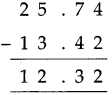(2) 206.35 – 168.22
Solution: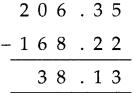(3) 63.4 – 31.8
Solution: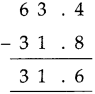(4) 63.43 – 31.8
Solution: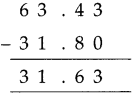(5) 63.4 – 31.83
Solution: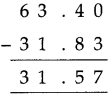(6) 8.23 – 5.45
Solution: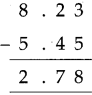(7) 18.23 – 9.45
Solution: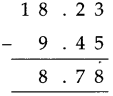(8) 78.03 – 41.65
Solution: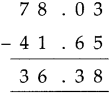Question 2.
Vrinda was 1.48 m tall. After a year, her height became 1.53 m. How many centimeters did her height increase in a year?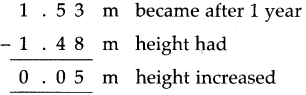∴ 5 cm height has increased in a year.

Something more

Decimals used for measurement

We need to measure distance, mass (weight) and volume every day. We use suitable units for these measurements. Kilometre, metre and centimeter for distance; litre, millilitre for volume and kilogram and gram for mass are the units that are used most of the time.All these units are decimal units. In this method, gram, metre and litre are taken as the basic units for mass, distance and volume respectively. Units larger than these increase 10 times at every step and smaller units become $$\frac{1}{10}$$ of the previous unit at each step.

Look at the table of these units given below.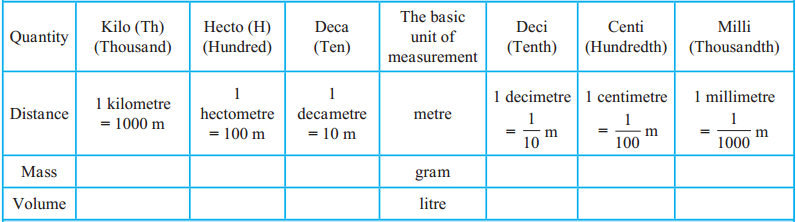The origin of the terms kilo, hecto… milli is in the Greek or Latin language. Their English equivalents are given in brackets along with the terms.

Subtract the following:

(1) 304.17 – 95.28
Solution: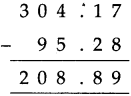(2) 72.84 – 36.96
Solution: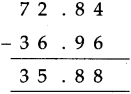(3) 9.17 – 5.88
Solution: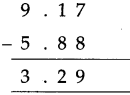(4) 100 – 49.99
Solution: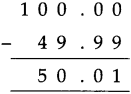(5) Atul has 56.25 and Anup has 65. Whose amount is more? How much?
Solution: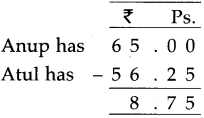∴ Anup’s amount is more by ₹ 8.75